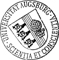Diffeomorphic Matching and Dynamic Deformable Surfaces in 3D Medical Imaging

• We consider optimal matching of submanifolds such as curves and surfaces by a variational approach based on Hilbert spaces of diffeomorphic transformations. In an abstract setting, the optimal matching is formulated as a minimization problem involving actions of diffeomorphisms on regular Borel measures considered as supporting measures of the reference and the target submanifolds. The objective functional consists of two parts measuring the elastic energy of the dynamically deformed surfaces and the quality of the matching. To make the problem computationally accessible, we use reproducing kernel Hilbert spaces with radial kernels and weighted sums of Dirac measures which gives rise to diffeomorphic point matching and amounts to the solution of a finite dimensional minimization problem. We present a matching algorithm based on the first order necessary optimality conditions which include an initial-value problem for a dynamical system in the trajectories describing the deformation ofWe consider optimal matching of submanifolds such as curves and surfaces by a variational approach based on Hilbert spaces of diffeomorphic transformations. In an abstract setting, the optimal matching is formulated as a minimization problem involving actions of diffeomorphisms on regular Borel measures considered as supporting measures of the reference and the target submanifolds. The objective functional consists of two parts measuring the elastic energy of the dynamically deformed surfaces and the quality of the matching. To make the problem computationally accessible, we use reproducing kernel Hilbert spaces with radial kernels and weighted sums of Dirac measures which gives rise to diffeomorphic point matching and amounts to the solution of a finite dimensional minimization problem. We present a matching algorithm based on the first order necessary optimality conditions which include an initial-value problem for a dynamical system in the trajectories describing the deformation of the surfaces and a final-time problem associated with the adjoint equations. The performance of the algorithm is illustrated by numerical results for examples from medical image analysis.• Dokument_1.pdfAuthor: Robert Azencott, Roland GlowinskiGND, Jiwen He, Ronald H. W. HoppeGND, Aarti Jajoo, Yipeng Li, Andrey Martynenko, Sagit Benzekry, Stephen H. Little, William A. Zoghbi urn:nbn:de:bvb:384-opus4-11502 https://opus.bibliothek.uni-augsburg.de/opus4/1390 Preprints des Instituts für Mathematik der Universität Augsburg (2010-07) Preprint English Universität Augsburg University of Houston, Ecole Normale Superieure Cachan, Medical Office Houston, The Methodist Hospital Research Institute 2010/04/27 diffeomorphic image matching; deformable surfaces; reproducing kernel Hilbert spaces; Dirac measures; medical image analysis Medizinische Informatik; Registrierung ; Hilbert-Raum; Matching-Problem; Dynamisches System; Anfangswertproblem Mathematisch-Naturwissenschaftlich-Technische Fakultät Mathematisch-Naturwissenschaftlich-Technische Fakultät / Institut für Mathematik Mathematisch-Naturwissenschaftlich-Technische Fakultät / Institut für Mathematik / Lehrstuhl für Numerische Mathematik 0 Informatik, Informationswissenschaft, allgemeine Werke / 00 Informatik, Wissen, Systeme / 004 Datenverarbeitung; InformatikDeutsches Urheberrecht mit Print on Demand